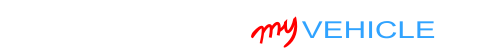## Interpreting Your Compression Test Results

For the most part, engine cylinders tend to wear evenly. But it's not uncommon in high-mileage engines, or engines that received little maintenance, to have uneven wear across the cylinders.

Up to a certain point, the uneven wear will not cause a problem and you won't notice any driveability problems.

It's only when the low compression cylinders are producing a value of less than 15% of the highest compression value (the engine is producing) that you'll have a misfire problem occurring in those cylinders.

To find out if the lower compression value is causing a problem, you need to find out if it's lower than 15% of the highest compression value you got.

You can do this (figuring out the 15%) in one of two ways: You can calculate this 15% difference with pen and paper or you can use my low compression calculator. You can find the low compression calculator here: Online Low Engine Compression Calculator (at: easyautodiagnostics.com).

If you want to manually calculate the 15% difference, here's what you'll need to do:

• STEP 1: Multiply the highest compression value by 0.15 (this is the decimal value of 15%).
• STEP 2: Round the result to the nearest one (for example: 25.6 would become 26).
• STEP 3: Subtract the result (the number that was rounded) from the highest compression value.
• ANSWER: The result of this subtraction is the lowest possible compression value any cylinder can have.

Now, let me give you a more specific example: Let's say that I got the following compression readings:

Cylinder Pressure
#1 165 PSI
#2   95 PSI
#3 155 PSI
#4 175 PSI
#5 175 PSI
#6 170 PSI
#7 175 PSI
#8 170 PSI

My next step is to do the following calculation:

• STEP 1:  175 x 0.15 = 26.25.
• STEP 2:  26.25 = 26 (rounded to nearest one).
• STEP 3:  175 - 26 = 149.
• ANSWER:  149 PSI. Any cylinder with this compression (or lower) value will misfire.

Since cylinder #2 is only producing 95 PSI, I can now conclude that it's 'dead' and causing a misfire.

To find out if the lowest compression value you got from your engine compression test is within a good range, you'll need to do the same calculation. Of course, you'll need to use the highest compression value you got and not the one in the example.

Once you've found the 'dead' cylinder, the next step is to find out what's causing the low compression value. For this step, go to: TEST 2: ‘Wet’ Engine Compression Test.

## TEST 2: ‘Wet’ Engine Compression Test

There is a way to find out if the low/zero compression value you got in TEST 1 is due to a problem in the cylinder's piston rings or in the cylinder's intake/exhaust valves.

And it simply involves adding about a tablespoon or two of engine oil to the cylinder with the low compression value.

The cylinder's compression is tested once again to see if the value increases or stays the same.

If the value increases, then you can conclude that the problem is due to bad piston rings. If the value does not increase, then you can conclude that the problem is in its cylinder head valves.

OK, this is what you need to do:

1. 1

Add a small amount of engine oil to the cylinder that reported low compression or no compression in the ‘Dry’ compression test.

You don't have to add a lot of oil. The amount should be about 1 to 2 tablespoons of oil.

2. 2

Install the compression tester onto the cylinder.

Do not use any type of tool to tightened the compression tester. Hand tight is fine.

3. 3

When all is set up, have your helper crank the engine.

4. 4

You'll get one of two results:

1.) The compression value will go up (from the one you recorded before).

2.) The compression value will stay the same.

Let's take a look at what your test results mean:

CASE 1: The compression value shot up. This tells you that the piston compression rings are worn out and thus the problem is in the bottom end (block) of the engine in your Chevrolet Express (GMC Savana).

CASE 2: The compression value stayed the same. This confirms that the low compression problem of the affected cylinder is due to worn or damaged cylinder head valves.

## More Chevrolet Express (GMC Savana) Tutorials

You can find a complete list of V8 Chevrolet Express (GMC Savana) tutorials in this index:

Here's a small sample of the tutorials you'll find in the index:If this info saved the day, buy me a beer!